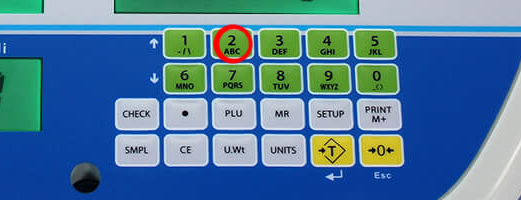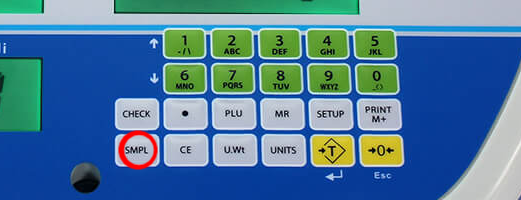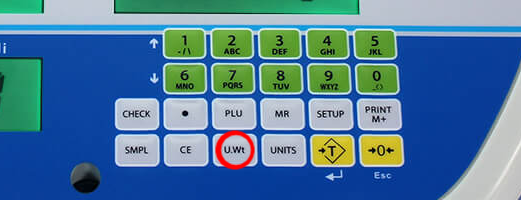Resource

#How to Count Pieces with a Counting Scale

Using a counting scale to count small pieces can save a lot of time. In this guide, we'll give you step by step instructions on counting pieces with a counting scale.

If you don't know the sample weight

A counting scale "counts" by weighing the samples and dividing the total weight by the individual weight. If you already know the piece weight, you can just input it into the scale and start counting. Here's how you find out the weight if you don't have it:

1. Place a container on the weighing pan
2. Tare the container using the tare button

3.Tare the container

4. Place the sample weight in the tared container. You can place a single sample, or a number of them if you know the exact quantity.
5. Once the sample is weighed, use the numeric keypad to enter the number of pieces you weighed.
6.Enter the number of pieces you weighed

7. Once the number of pieces is entered, press the SMPL key. This will save the number of pieces you entered, and the scale will calculate the weight of individual pieces if necessary.
8.Press the SMPL key to save the number of pieces you weighed

9. The 3 displays are labeled. You have the weight, which is the total weight on the scale (minus anything you tared). The unit weight is the individual weight of each piece. The piece count is the number of pieces on the scale.
10. You can now place the pieces on the scale, and it will count them, and give you the total weight. The unit weight might change as the scale refines the exact weight of the sample.

If you already know the unit weight, here's what you should do instead of steps 3-5:

1. Enter the weight with the numeric keypad.
2. Once you have entered the weight, press the key "U.Wt". This will save the weight you entered as the unit weight, and this number will be used to count the rest of the pieces.
3.Press the U.Wt key to save your weight as a unit weight

4. Follow steps 6 and 7 above.